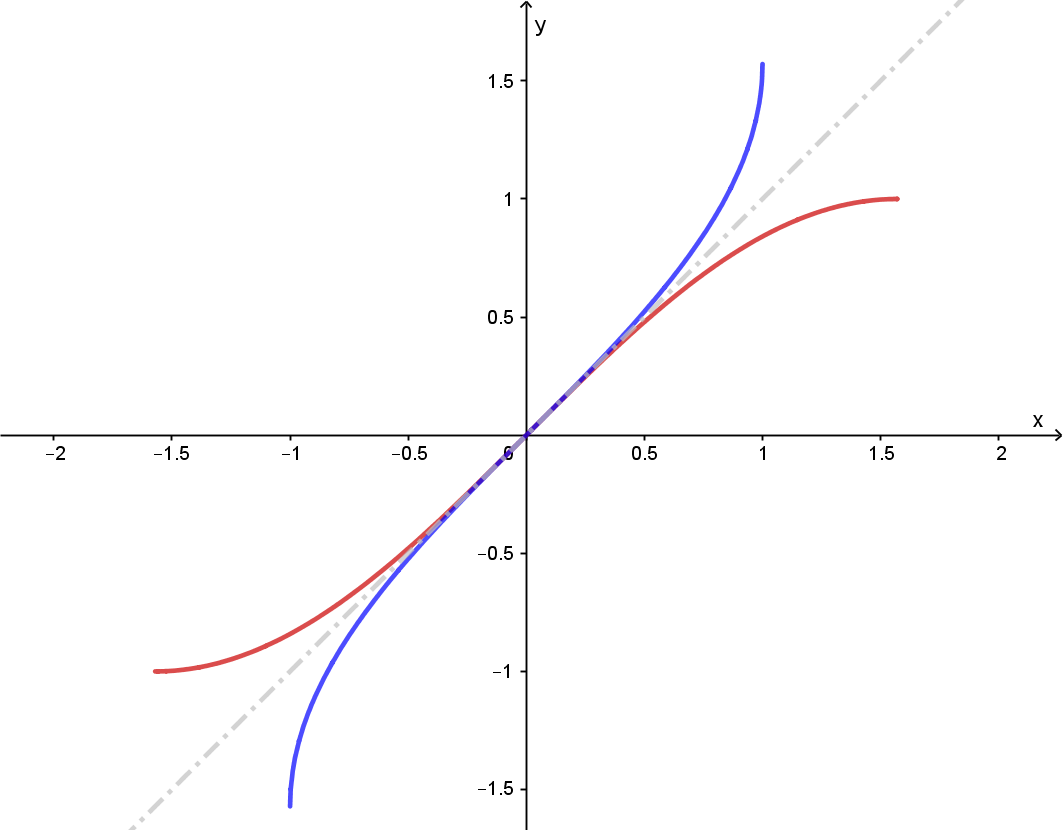# Section 1.7 Inverse Trigonometric Functions

Section Objectives

1. Define the inverse trigonometric functions.
2. Evaluate, graph, and use the inverse trigonometric functions.
3. Evaluate compositions of trig and inverse trig functions.

### Inverse Trig Functions

If a function passes the horizontal line test, then it has an inverse function. If an inverse exists, then it "undoes" what the original function does.

For example, if we restrict our attention to $x\ge0$, then $y=x^2$ and $y=\sqrt{x}$ are inverses. One undoes what the other does.

Another example is the pair $y=x+5$ and $y=x-5$. One adds 5; the other subtracts 5.

Because all of the trig functions are periodic, none of them pass the horizontal line test...unless we make restrictions on their domains. For the purposes of defining the inverses of the trig functions, we will restrict each function to the domain shown below:

• $y=\sin x, \quad -\pi/2 \le x \le \pi/2$
• $y=\cos x, \quad 0 \le x \le \pi$
• $y=\tan x, \quad -\pi/2 < x < \pi/2$
• $y = \csc x, \quad -\pi/2 \le x \le \pi/2, \, x \ne 0$
• $y = \sec x, \quad 0 \le x \le \pi, \, x \ne \pi/2$
• $y=\cot x, \quad 0 < x < \pi$

On the given interval, each function is one-to-one (the graph passes the horizontal line test) and has an inverse. Each inverse is denoted by using the "arc" prefix or a floating $-1$ suffix (not an exponent!):

The inverse of $\mbox{trig}(x)$ is denoted $\mbox{arctrig}(x)$ or $\mbox{trig}^{-1}(x)$, where by "trig" we mean any one of the trigonometric functions.

Each inverse trig function is defined by the action of undoing the corresponding trig function.

For example, $x = \sin y$ if and only if $\sin^{-1} x = y$. The arcsine function undoes the sine function, and vice versa!

Here is a fairly easy way to think about $\mbox{trig}^{-1}(x)$:

$\mbox{trig}^{-1}(x)$ is the number (in the correct range of values) whose trig function value is $x$

It is important to note that the output values of an inverse function are the input values of the original function. Similarly, the input values of an inverse are the outputs of the original.

#### Examples

• $\displaystyle \sin^{-1} \frac{1}{2} = \frac{\pi}{6} \quad$ because $\quad \displaystyle \sin \frac{\pi}{6} = \frac{1}{2}$. Or equivalently, $\displaystyle \sin^{-1} \frac{1}{2} - \frac{\pi}{6}$ because $\pi/6$ is the angle in $[\pi/2,\pi/2]$ whose sine is $1/2$.

• Compute $\displaystyle \arcsin \frac{\sqrt{2}}{2}$

• Compute $\sin^{-1} 1$

• Compute $\displaystyle \arccos \frac{1}{2}$

• Compute $\tan^{-1}(1)$ and $\tan^{-1} (-1)$

• Compute $\displaystyle \csc^{-1} \left( -\frac{2}{\sqrt{3}} \right)$

Important note: For now, use your unit circle to help you with familiar angles. But make sure you always use the correct range of values.

### Graphs

The graphs of the inverse trig functions can be obtained by flipping the graph of the corresponding trig function (appropriately restricted!) about the line $y=x$.

For example, here is the graph of $y=\sin x$ and $y=\sin^{-1} x$.This "flipping" also flips any asymptotes. What do you think the graph of $y=\tan^{-1} x$ looks like?

### Compositions of Trig and Inverse Trig Functions

When functions are inverses, they "undo" each other. Therefore, we should expect that

$\mbox{trig}(\mbox{trig}^{-1}(x))=x$ and $\mbox{trig}^{-1}(\mbox{trig}(x))=x$.

This is mostly true...but we must be very careful about using the correct ranges of values. Inverse properties do not apply for arbitrary values!

#### Examples

• Compute $\displaystyle \arcsin \left( \sin \frac{3\pi}{2} \right)$

• Compute $\displaystyle \tan \left( \arctan(-5) \right)$

• Compute $\displaystyle \arcsin \left( \sin \frac{5\pi}{3} \right)$

It is an interesting, and very useful, fact that the composition of a trig function and an inverse trig function (in that order) can be simplified with the use of a right triangle. This idea is illustrated with the following examples...

• Find the exact value of $\tan( \arccos(2/3))$

• Find the exact value of $\cos( \sin^{-1} (-3/5))$

• Simplify $\sin(\cos 3x)$ if $0 \le x \le 1/3$